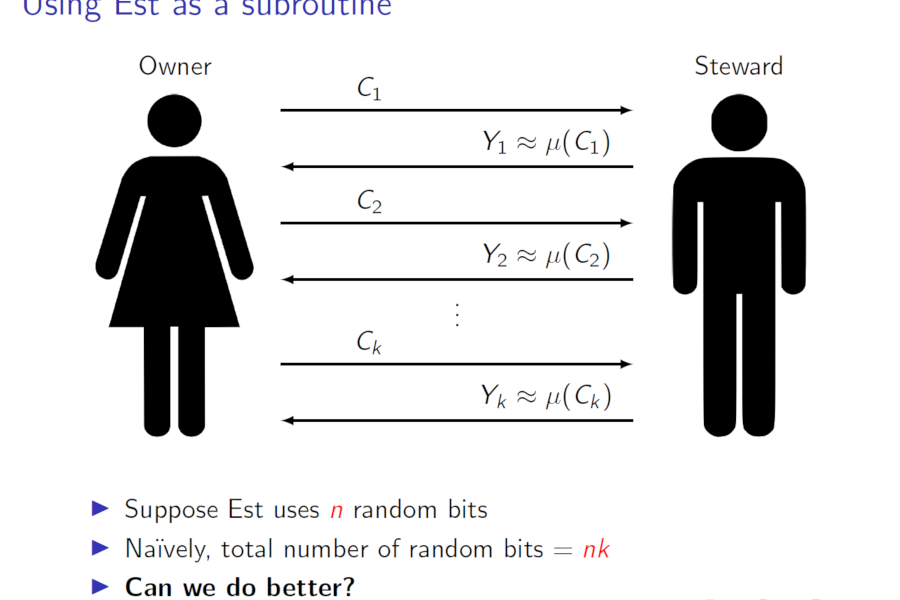Back to list of papers

# Preserving Randomness for Adaptive Algorithms

By William M. Hoza and Adam R. Klivans

Abstract (for specialists)

Suppose $$\mathsf{Est}$$ is a randomized estimation algorithm that uses $$n$$ random bits and outputs values in $$\mathbb{R}^d$$. We show how to execute $$\mathsf{Est}$$ on $$k$$ adaptively chosen inputs using only $$n + O(k \log(d + 1))$$ random bits instead of the trivial $$nk$$ (at the cost of mild increases in the error and failure probability). Our algorithm combines a variant of the INW pseudorandom generator (STOC '94) with a new scheme for shifting and rounding the outputs of $$\mathsf{Est}$$. We prove that modifying the outputs of $$\mathsf{Est}$$ is necessary in this setting, and furthermore, our algorithm's randomness complexity is near-optimal in the case $$d \leq O(1)$$. As an application, we give a randomness-efficient version of the Goldreich-Levin algorithm; our algorithm finds all Fourier coefficients with absolute value at least $$\theta$$ of a function $$F \colon \{0, 1\}^n \to \{-1, 1\}$$ using $$O(n \log n) \cdot \text{poly}(1/\theta)$$ queries to $$F$$ and $$O(n)$$ random bits (independent of $$\theta$$), improving previous work by Bshouty et al. (JCSS '04).

Not-so-abstract (for curious outsiders)

⚠️ This summary might gloss over some important details.

Suppose you have a randomized algorithm that estimates some numeric quantity, and you plan to use it $$k$$ times. Suppose the estimation algorithm makes $$n$$ coin tosses. This situation seems to call for $$nk$$ coin tosses in total. In this paper, we show that you can actually get away with only making about $$n + k$$ coin tosses if you use some tricks.

We posted a manuscript online in November 2016; I presented the paper at RANDOM in August 2018. The arXiv version and the ECCC version are identical. The RANDOM proceedings version is more compact and skips a lot of the material.

Expository material:Slides from my presentation at RANDOM (August 2018). See also these slides from my longer presentation at Caltech's CS Theory Seminar (May 2017). In both cases, I recommend viewing the slides in Adobe Reader to get the animations to work.

Video from Adam's presentation at the Simons Institute "Proving and Using Pseudorandomness" workshop (March 2017).